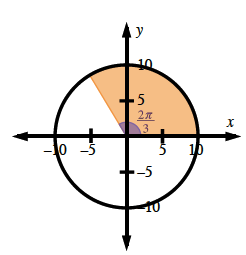Home > A2C > Chapter 8 > Lesson 8.1.5 > Problem8-85

8-85.
1. Sketch a graph of x2 + y2 = 100. Homework Help ✎

1. Is it a function?

2. What are its domain and range?

3. Draw a central angle that measures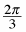radians. If you remove this wedge of the circle, how much area remains?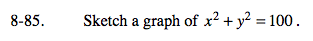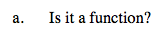Does each x-value on the graph of the function have only one corresponding y-value?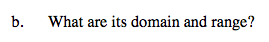Domain: −10 ≤ x ≤ 10
Range: −10 ≤ y ≤ 10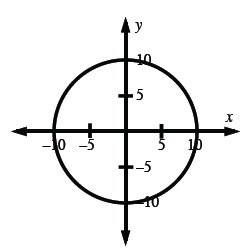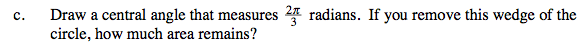See the unit circle graph at right.
What portion of the circle is shaded?

$\text{Since}\:\frac{2\pi}{3}\:\text{is}\:\frac{1}{3}\:\text{of\:the\:total\:angle\:in\:a\:circle,\:the\:remaining}$

$\text{area\:will\:be\:}\frac{2}{3}\:\text{of\:the\:total\:area.}$